# waynehu

## Professor, Department of Astronomy and Astrophysics University of Chicago

Group Contact CV SnapShots

## From Anisotropies to Polarization

The Thomson scattering cross section depends on polarization as (see e.g. [Chandrasekhar] 1960)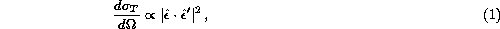where(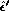) are the incident (scattered) polarization directions. Heuristically, the incident light sets up oscillations of the target electron in the direction of the electric field vector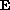, i.e. the polarization. The scattered radiation intensity thus peaks in the direction normal to, with polarization parallel to, the incident polarization. More formally, the polarization dependence of the cross section is dictated by electromagnetic gauge invariance and thus follows from very basic principles of fundamental physics.

If the incoming radiation field were isotropic, orthogonal polarization states from incident directions separated by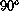would balance so that the outgoing radiation would remain unpolarized. Conversely, if the incident radiation field possesses a quadrupolar variation in intensity or temperature (which possess intensity peaks at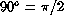separations), the result is a linear polarization of the scattered radiation (see Fig. 1). A reversal in sign of the temperature fluctuation corresponds to arotation of the polarization, which reflects the spin-2 nature of polarization.

Fig. 1: Thomson scattering of radiation with a quadrupole anisotropy generates linear polarization. Blue colors (thick lines) represent hot and red colors (thin lines) cold radiation.

In terms of a multipole decomposition of the radiation field into spherical harmonics,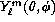, the five quadrupole moments are represented by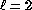,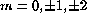. The orthogonality of the spherical harmonics guarantees that no other moment can generate polarization from Thomson scattering. In these spherical coordinates, with the north pole at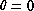, we call a N-S (E-W) polarization component Q>0 (Q<0) and a NE-SW (NW-SE) component U>0 (U<0). The polarization amplitude and angle clockwise from north are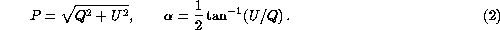Alternatively, the Stokes parameters Q and U represent the diagonal and off diagonal components of the symmetric, traceless,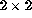intensity matrix in the polarization plane spanned by (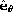,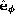),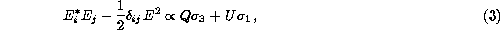where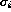are the Pauli matrices and circular polarization is assumed absent.

If Thomson scattering is rapid, then the randomization of photon directions that results destroys any quadrupole anisotropy and polarization. The problem of understanding the polarization pattern of the CMB thus reduces to understanding the quadrupolar temperature fluctuations at last scattering.

Temperature perturbations have 3 geometrically distinct sources: the scalar (compressional), vector (vortical) and tensor (gravitational wave) perturbations. Formally, they form the irreducible basis of the symmetric metric tensor. We shall consider each of these below and show that the scalar, vector, and tensor quadrupole anisotropy correspond torespectively. This leads to different patterns of polarization for the three sources as we shall discuss in §3.

Next: Scalar Perturbations